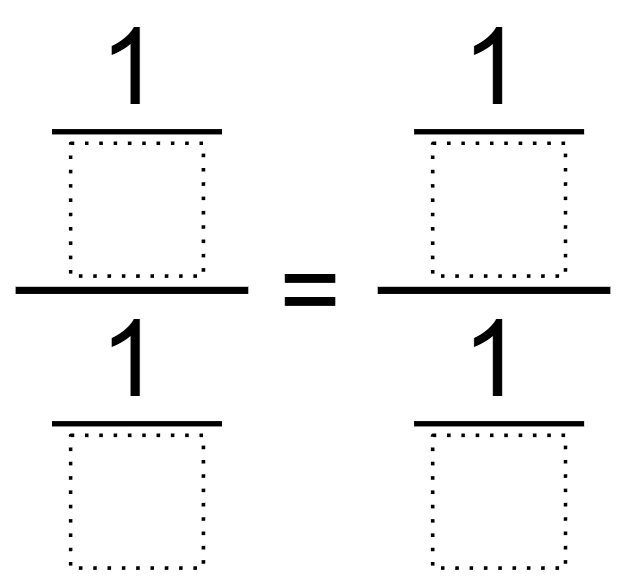# Unit Fraction Proportion

Directions: Fill in the boxes using the digits 1 through 9, at most one time each, to make a true equality statement### Hint

What makes equivalent Ratios? How does that relate to fractions?

There are multiple correct answers, one example would be (1/2)/(1/4)= (1/3)/(1/6)

Source: Bryan Anderson

## Two Step Inequality with Fractional Coeffcient

Directions: Using the digits 1 to 7 at most one time each, place a digit …

1.there are multiple correct answer, one example woulds be (1/2)/(1/4)=(1/3)/(1/6).

2.3.4.5.6.